# Tweets by @WolframResearch

Data used in the Wolfram U interactive course “Multiparadigm Data Science”

## Details

The following information is available for each tweet in the dataset: {ID, Text, Date, Hashtags, Location, Username, Name, ProfileImageThumbnail, RetweetCount, FavoriteCount, URL}

## Examples

### Basic Examples

Retrieve the resource:

 In:=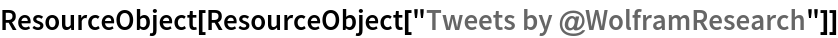Out=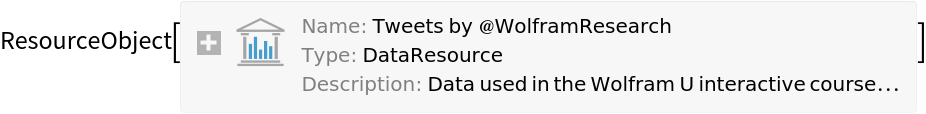Retrieve the default content:

 In:=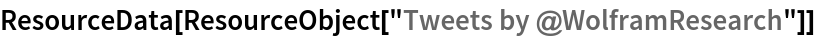Out=Retrieve user data for the users mentioned in the tweets by WolframResearch:

 In:=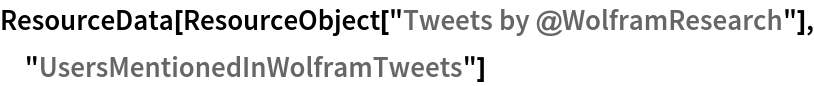Out=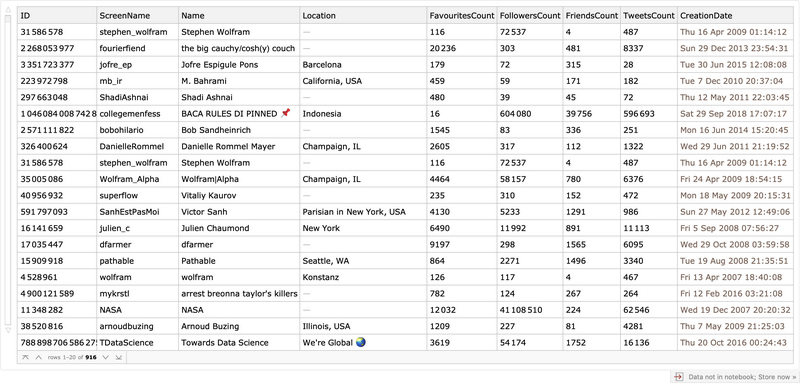Retrieve locations of the users mentioned in the tweets by WolframResearch (3 random locations):

 In:=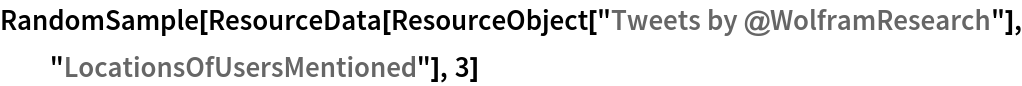Out=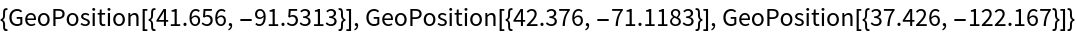Retrieve hashtags used by the users mentioned in the tweets by WolframResearch (3 random users):

 In:=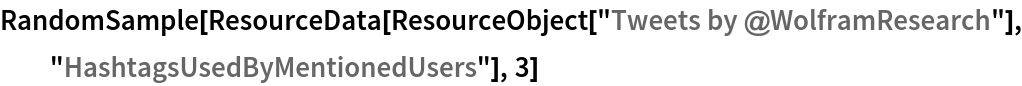Out=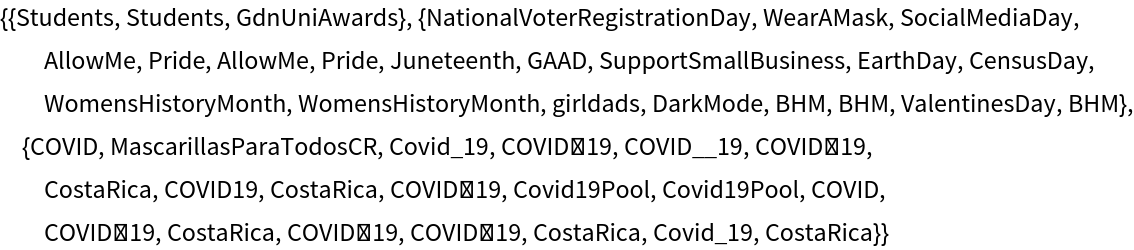Retrieve a graph of users mentioning each other (3 random edges):

 In:=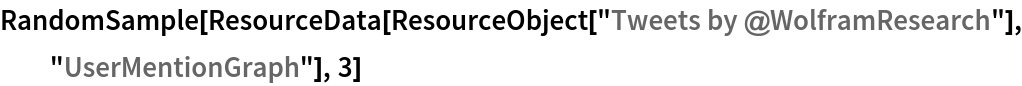Out=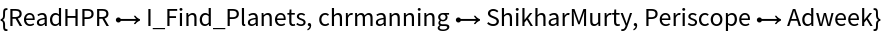### Visualizations

Visualize a DateHistogram of tweets by Wolfram research:

 In:=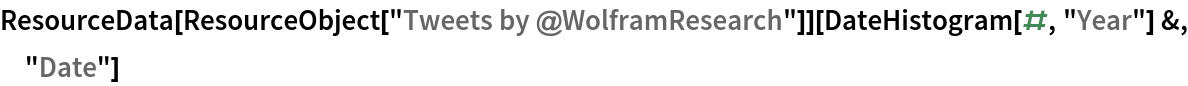Out=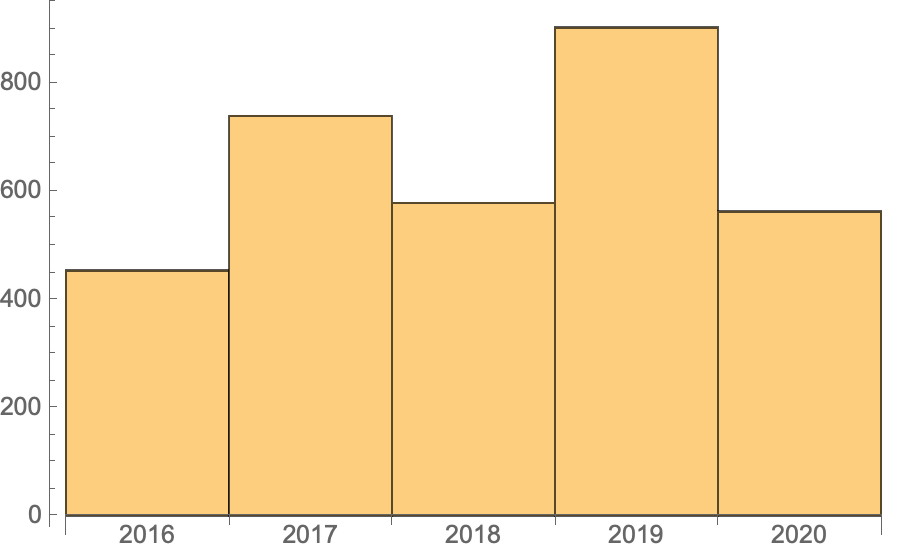Visualize a WordCloud of other users mentioned in the tweets by WolframResearch:

 In:=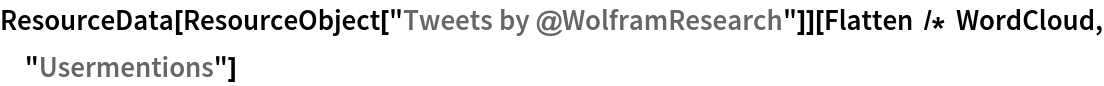Out=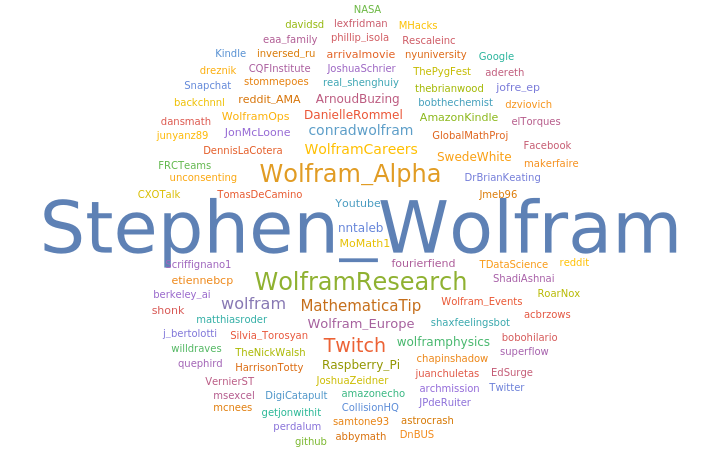Visualize the locations of the users mentioned in the tweets by WolframResearch:

 In:=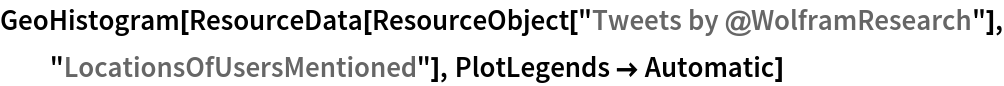Out=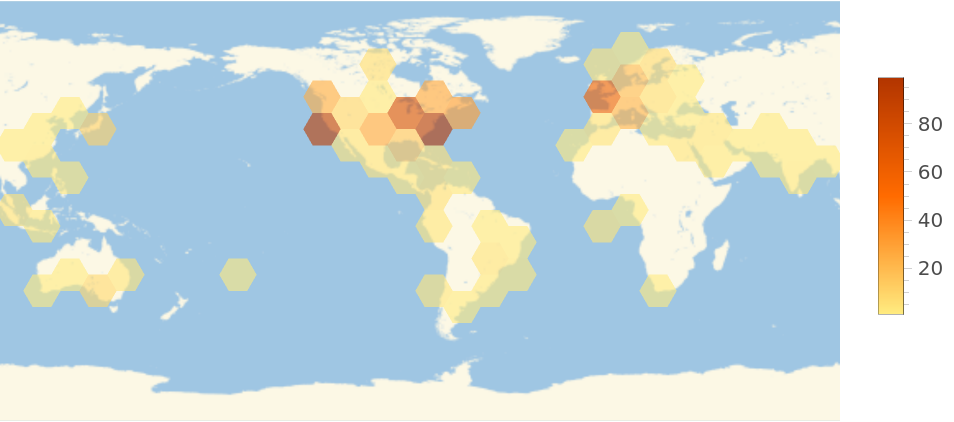Visualize a WordCloud of the hashtags used by these users:

 In:=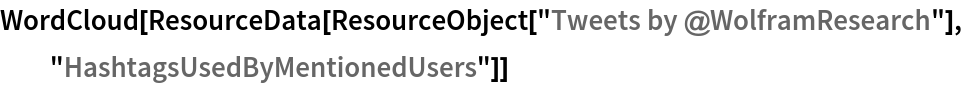Out=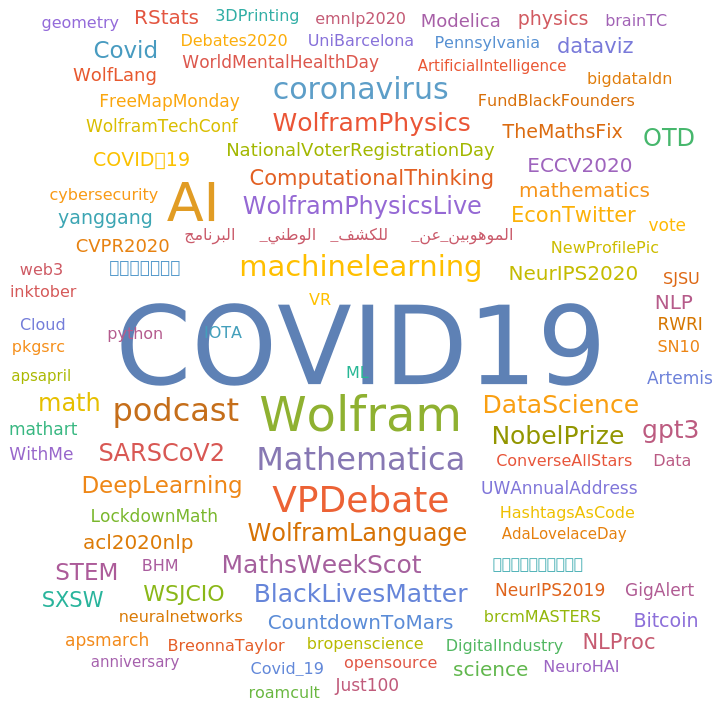### Analysis

Visualize users mentioning each other in their tweets in a graph:

 In:=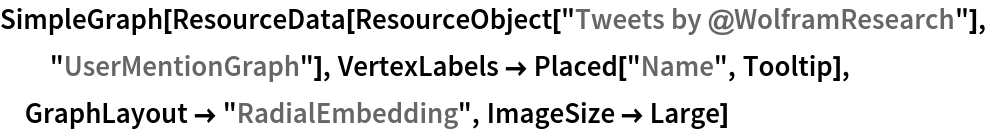Out=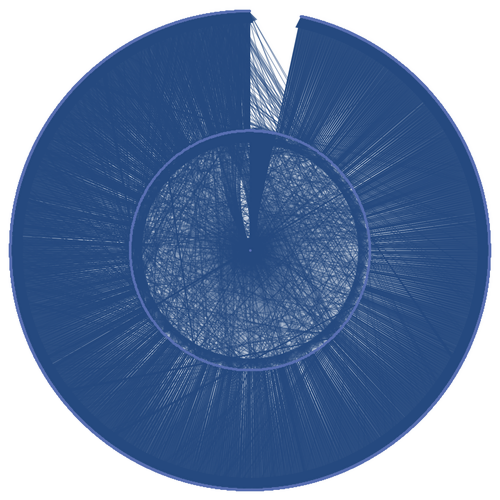Wolfram U, "Tweets by @WolframResearch" from the Wolfram Data Repository (2020)